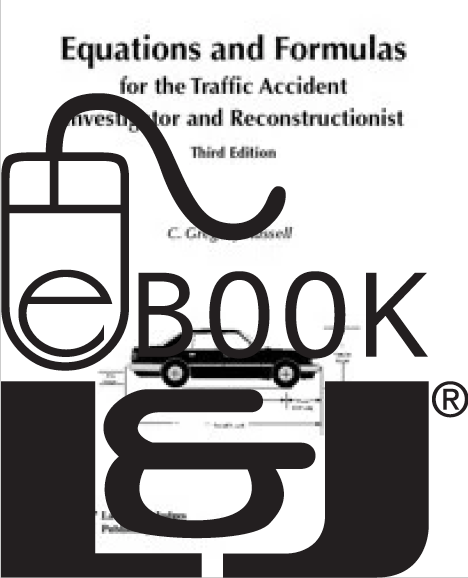Lawyers & Judges Publishing Company, Inc.# Equations & Formulas for the Traffic Accident Investigator and Reconstructionist, Third Edition PDF eBook

\$ 49.00

• Author: C. Gregory Russell
• ISBN 10: 1-936360-23-3
• ISBN 13: 978-1-936360-23-9
• Copyright Date Ed:  January 27, 2014
• Pages: 224 pages
• PDF eBook

Over 200 must-have accident reconstruction formulas at your fingertips in this revised Third Edition.

This unique resource is designed to provide, in an easy to use format, the majority of the equations needed for accident reconstruction and investigation. Designed for flexibility and ease of use, each equation is expressed in three formats: algebraic; modified long form; and spreadsheet format. Formulas and constants for converting between metric and imperial units are provided for worldwide use.

## Topics Include

• Acceleration/Deceleration
• Kinetic Energy
• Speed
• Velocity
• Distance
• Time
• Falls
• Vaults
• Flips
• Conservation of Momentum
• Stiffness Coefficients
• Crush Damage/Energy
• Equivalent Barrier Speed
• Restitution
• Principle Direction of Force
• Center of Gravity
• Rollover Speed
• Offtracking
• Specialized Equations for Pedestrian and Bicycle Accidents
• And more!

Information and Equations included:

Newton’s Laws of Motion
2xg versus 30 and 254
Acceleration/Deceleration Factor
Braking Efficiency
Acceleration Rate
Kinetic Energy
Basic Speed/Slide to Stop/Minimum Speed Equation
Combined Speed/Velocity Equations
Speed/Velocity From Kinetic Energy
Converting Speed to and From Velocity
Critical Speed/Velocity
Speed of Hydroplane
Velocity Equations
Distance Equations
Time Equations
Falls
Vaults
Flips
Conservation of Momentum — In-Line Collisions
Conservation of Momentum — 360°
Stiffness Coefficients
Average Crush Depth
Damage Centroid Location
Crush/Damage Energy
Average Crush Depth from Crush Damage Energy
Equivalent Barrier Speed in Pole Impacts
Dissipation of Energy
ov From Vehicle Velocities
Vehicle Velocities from ov
ov From Vehicle Damage (Crush)
Restitution
Principle Direction of Force (pdof)
Moment Arm Length
Pedestrian Equations
Fall and Slide based Pedestrian Equations
Miscellaneous Pedestrian Equations
Bicycle Equations
Speed/Velocity From Weight Shift
Center of Gravity
Rollover Speed Propensity
Offtracking
Miscellaneous Equations
Alcohol Levels
Trigonometry
Law of Sines
Law of Cosines
Geometry
Mathematical Order of Operation
Derivations
360°Angular Momentum — Solving for V2
360°Angular Momentum — Solving for V1
Kinetic Energy .
Speed and Velocity form Kinetic Energy
Basic Speed or Slide to Stop Equation
Combined Speed Equation